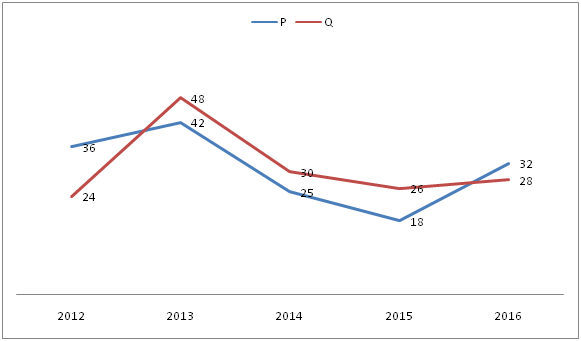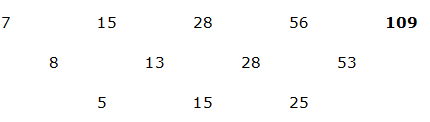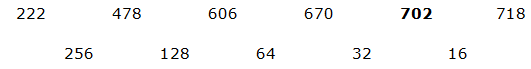# NIACL AO Prelims – Quantitative Aptitude Questions Day- 83

Dear Readers, Bank Exam Race for the Year 2019 is already started, To enrich your preparation here we have providing new series of Practice Questions on Quantitative Aptitude – Section. Candidates those who are preparing for NIACL AO Prelims 2019 Exams can practice these questions daily and make your preparation effective.

[WpProQuiz 4970]

Directions (1 – 5): Find the wrong number in the given series.

1) 1278, 1279, 1306, 1431, 1775

a) 1278

b) 1279

c) 1306

d) 1775

e) 1431

2) 7, 15, 28, 56, 110

a) 7

b) 110

c) 15

d) 28

e) 56

3) 15, 16, 34, 105, 425, 2125

a) 425

b) 105

c) 34

d) 16

e) 15

4) 222, 478, 606, 670, 700, 718

a) 478

b) 606

c) 700

d) 718

e) 670

5) 3, 9, 42, 261, 2100, 21010

a) 21010

b) 261

c) 2100

d) 42

e) 9

Directions (6 – 10): Study the following information carefully and answer the given questions:

The following graph shows the total no of bags (in thousands) sold by two stores in different years.6) What is the ratio between the total no of bags sold by store P in all the given years together to that in the total no of bags sold by store Q in all the given years together?

a) 51 : 52

b) 41 : 43

c) 38 : 25

d) 27 : 24

e) None of these

7) In 2015, 30 % of bags sold by Store P and 25 % of the bags sold by store Q were leather bags. What was the total no of leather bags sold by store P and Q together in 2015?

a) 9600

b) 11900

c) 11200

d) 12800

e) None of these

8) What is the ratio of total no of bags sold by Store P and Q together in 2013 to that in 2016?

a) 4 : 3

b) 5 : 6

c) 7: 5

d) 3: 2

e) None of these

9) Total no of bags sold by Store P in the year 2012, 2014 and 2016 together is approximately what percentage of total no of bags sold by Store Q in the year 2013, 2014 and 2015 together?

a) 89 %

b) 94 %

c) 76 %

d) 103 %

e) 118 %

10) If the average no of bags sold by Store Q in the year 2013 to 2017 is 33 thousands, then find the total no of bags sold by Store Q in the year 2017? (In thousands)

a) 45

b) 51

c) 33

d) 27

e) None of these

Direction (1-5) :

1278 + 13 = 1279

1279 + 33 = 1306

1306 + 53 = 1431

1431 + 73 = 177415 * 1 + 1 = 16

16 * 2 + 2 = 34

34 * 3 + 3 = 105

105 * 4 + 4 = 424

424 * 5 + 5 = 2125256/2 = 128

128/2 = 64

64/2 = 32

32/2 = 16

3 * 2 + 3 = 9

9 *4 + 6 = 42

42 *6 + 9 = 261

261 * 8 + 12 = 2100

2100 * 10 + 15 = 21015

Direction (6-10) :

The total no of bags sold by store P in all the given years together

= > (36 + 42 + 25 + 18 + 32) thousands

= > 153 thousands

The total no of bags sold by store Q in all the given years together

= > (24 + 48 + 30 + 26 + 28) thousands

= > 156 thousands

Required ratio = 153: 156 = 51: 52

In 2015,

Leather bags in store P = 18000*(30/100) = 5400

Leather bags in store Q = 26000*(25/100) = 6500

Total no of leather bags in Store P and Q in 2015 = 5400 + 6500 = 11900

The total no of bags sold by Store P and Q together in 2013

= > 48000 + 42000 = 90000

The total no of bags sold by Store P and Q together in 2016

= > 28000 + 32000 = 60000

Required ratio = 90000: 60000 = 3: 2

Total no of bags sold by Store P in the year 2012, 2014 and 2016 together

= > (36 + 25 + 32) thousand = 93000

Total no of bags sold by Store Q in the year 2013, 2014 and 2015 together

= > (48 + 30 + 26) thousand = 104000

Required % = (93000/104000)*100 = 89.42 % = 89 %

The average no of bags sold by Store Q in the year 2013 to 2017 = 33 thousands

The total no of bags sold by Store Q in the year 2013 to 2017 = 33*5 = 165 thousands

The total no of bags sold by Store Q in the year 2013 to 2016 = 132 thousands

The total no of bags sold by Store Q in the year 2017 = 165 – 132 = 33 thousands